# Introduction to GANs (Generative Adversarial Networks)

0
1187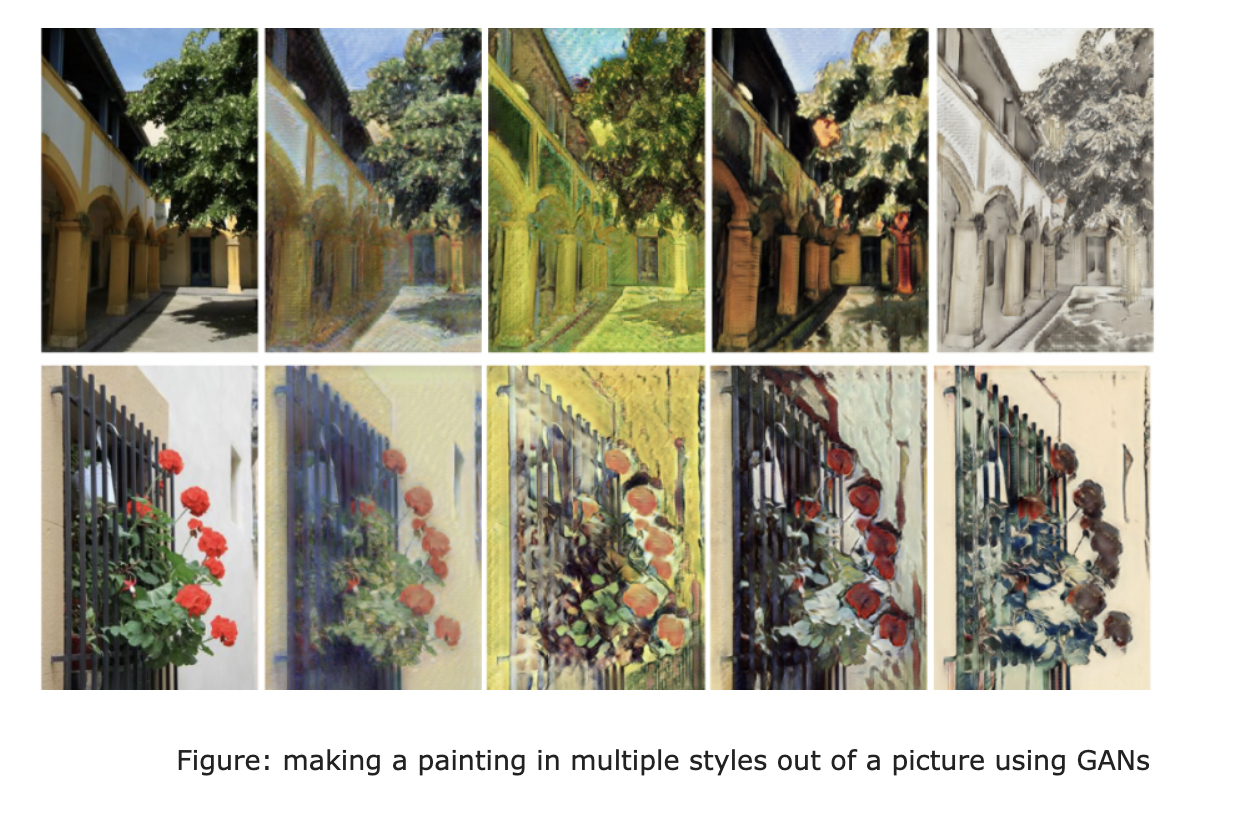Generative Adversarial Networks, or GANs, belong to generative models, which create new data instances that resemble the training data. GANs are algorithmic architectures that make use of two neural networks, a generative model G that evaluates the data distribution, and a discriminative model D that calculates the probability that a sample came from the training data rather than G. to generate new, synthetic instances of data that can pass for actual data, The generator tries to fool the Discriminator. The Discriminator tries to keep from being deceived.GANs are rapidly transforming the field and are used widely in image generation, video generation, and voice generation.

Generative models tackle a more formidable task than discriminative models as Discriminative models only draw boundaries in the data space. The discriminative model tries to tell the difference between data clusters by drawing a line between them in the data space. It can distinguish between the sets without ever having to model exactly where the instances are placed. In contrast, the generative model tries to produce convincing data instances by generating points that fall close to their natural counterparts in the data space.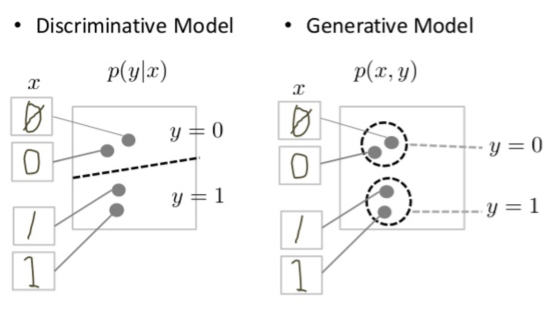Figure: Discriminative and generative models of handwritten digits.

Generative modeling is an unsupervised learning task. Unsupervised Learning involves automatically discovering information and learning the input data patterns to generate new examples without any supervision. Supervised Learning consists of training the machine using data that is already tagged with the correct answer. A supervised learning algorithm learns from labeled training data and predicts outcomes.

The Discriminator

The Discriminator in a GAN is simply a classifier that distinguishes actual data from fake data, i.e., data created by the generator. The Discriminator’s training data comes from Real data instances, which are used as positive examples during training. Fake data instances created by the generator are used as negative examples during training.

The Generator

The generator in a GAN generates fake samples of data and tries to fool the Discriminator. It learns to make the Discriminator classify its output as real. The generator’s training includes random input, a discriminator network, classifying the generated data, and generator loss, penalizing the generator for failing to fool the Discriminator.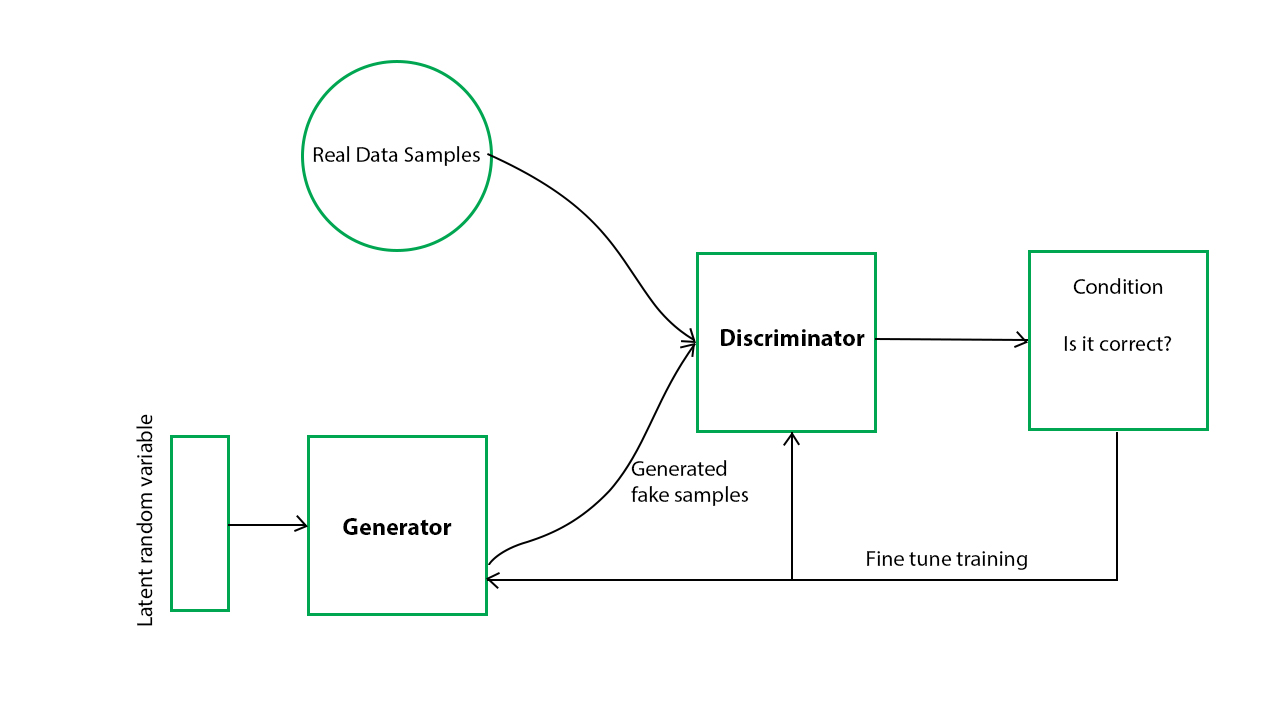source: geeksforgeeks

How GANs Work

The generator generates new data instances, while the Discriminator decides whether each sample of data that it evaluates belongs to the actual training dataset or not. The goal of the Discriminator, when shown a sample from the actual dataset, is to recognize those that are authentic. Meanwhile, the generator creates new, synthetic images that are passed to the Discriminator in the hopes that they, too, will be deemed original, even though they are fake.

So, the generator takes in random numbers and returns a picture. This generated picture is fed into the Discriminator alongside pictures taken from the actual, ground-truth dataset. The Discriminator takes in both pictures and returns a number between 0 and 1, with 1 representing authenticity and 0 representing fake. The Discriminator is in a feedback loop with the ground truth of the pictures, and the generator is in a feedback loop with the Discriminator.

GAN training proceeds in alternating periods. The generator is constant during the discriminator training phase as Discriminator has to learn how to recognize the generator’s flaws. Similarly, the Discriminator is constant during the generator training phase to make the training processes converge. As the generator improves with training, the Discriminator’s performance worsens because it can’t quickly tell the difference between real and fake. If the generator succeeds, then the Discriminator has only 50% accuracy.

Building a Digit Generator with GANs

Now that we understand GANs let’s use them to produce a clone of a random digit. We will train the models using the MNIST dataset of handwritten digits and use Keras to generate the model.

Write the following code to implement the model:

``````from keras.datasets import mnist
from keras.layers import *
from keras.layers.advanced_activations import LeakyReLU
from keras.models import Sequential, Model
from keras.optimizers import Adam

import numpy as np
import matplotlib.pyplot as plt
import math

# Load and Normalize this data [-1,1]
(X_Train,_),(_,_) = mnist.load_data()
X_Train  = (X_Train.astype('float32') - 127.5)/127.5

TOTAL_EPOCHS = 50
BATCH_SIZE = 256
NO_OF_BATCHES = int(X_Train.shape/BATCH_SIZE)
HALF_BATCH = 128
NOISE_DIM = 100 # Upsample into 784 Dim Vector
adam = Adam(lr=2e-4,beta_1=0.5)

# Generator
# Input Noise (100 dim) and Outputs a Vector (784 dim)

generator = Sequential()
generator.add(Dense(256,input_shape=(NOISE_DIM,)))
generator.add(LeakyReLU(0.2))
generator.add(Dense(512))
generator.add(LeakyReLU(0.2))
generator.add(Dense(1024))
generator.add(LeakyReLU(0.2))
generator.add(Dense(784,activation='tanh'))
generator.compile(loss='binary_crossentropy',optimizer=adam)

# Discriminator
discriminator = Sequential()
discriminator.add(Dense(512,input_shape=(784,)))
discriminator.add(LeakyReLU(0.2))
discriminator.add(Dense(256))
discriminator.add(LeakyReLU(0.2))
discriminator.add(Dense(1,activation='sigmoid'))
discriminator.compile(loss='binary_crossentropy',optimizer=adam)

# GAN (Step-2)
discriminator.trainable = False
gan_input = Input(shape=(NOISE_DIM,))
generated_img = generator(gan_input)
gan_output = discriminator(generated_img)

# Functional API
model = Model(gan_input,gan_output)
model.compile(loss='binary_crossentropy',optimizer=adam)

X_Train = X_Train.reshape(-1,784)

def save_imgs(epoch,samples=100):

noise = np.random.normal(0,1,size=(samples,NOISE_DIM))
generated_imgs = generator.predict(noise)
generated_imgs = generated_imgs.reshape(samples,28,28)

plt.figure(figsize=(10,10))
for i in range(samples):
plt.subplot(10,10,i+1)
plt.imshow(generated_imgs[i],interpolation='nearest',cmap='gray')
plt.axis("off")

plt.tight_layout()
plt.savefig('images/gan_output_epoch_{0}.png'.format(epoch+1))
plt.show()

# Training Loop
d_losses = []
g_losses = []

for epoch in range(TOTAL_EPOCHS):
epoch_d_loss = 0.
epoch_g_loss = 0.

#Mini Batch SGD
for step in range(NO_OF_BATCHES):

# Step-1 Discriminator
# 50% Real Data + 50% Fake Data

#Real Data X
idx = np.random.randint(0,X_Train.shape,HALF_BATCH)
real_imgs = X_Train[idx]

#Fake Data X
noise = np.random.normal(0,1,size=(HALF_BATCH,NOISE_DIM))
fake_imgs = generator.predict(noise) #Forward

# Labels
real_y = np.ones((HALF_BATCH,1))*0.9 #One Side Label Smoothing for Discriminator
fake_y = np.zeros((HALF_BATCH,1))

# Train our Discriminator
d_loss_real = discriminator.train_on_batch(real_imgs,real_y)
d_loss_fake = discriminator.train_on_batch(fake_imgs,fake_y)
d_loss = 0.5*d_loss_real + 0.5*d_loss_fake

epoch_d_loss += d_loss

# Train Generator (Considering Frozen Discriminator)
noise = np.random.normal(0,1,size=(BATCH_SIZE,NOISE_DIM))
ground_truth_y = np.ones((BATCH_SIZE,1))
g_loss = model.train_on_batch(noise,ground_truth_y)
epoch_g_loss += g_loss

print("Epoch %d Disc Loss %.4f Generator Loss %.4f" %((epoch+1),epoch_d_loss/NO_OF_BATCHES,epoch_g_loss/NO_OF_BATCHES))
d_losses.append(epoch_d_loss/NO_OF_BATCHES)
g_losses.append(epoch_g_loss/NO_OF_BATCHES)

if (epoch+1)%5==0:
generator.save('model/gan_generator_{0}.h5'.format(epoch+1))
save_imgs(epoch)
``````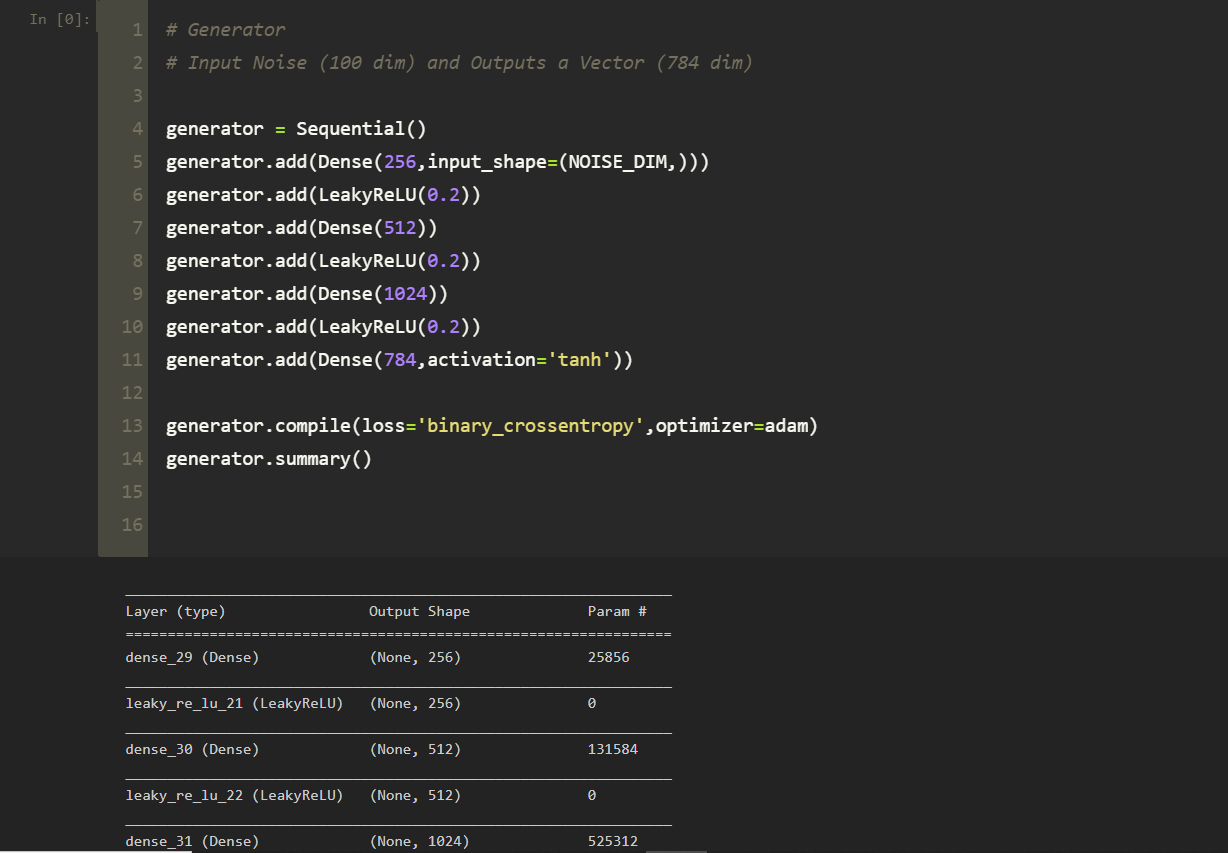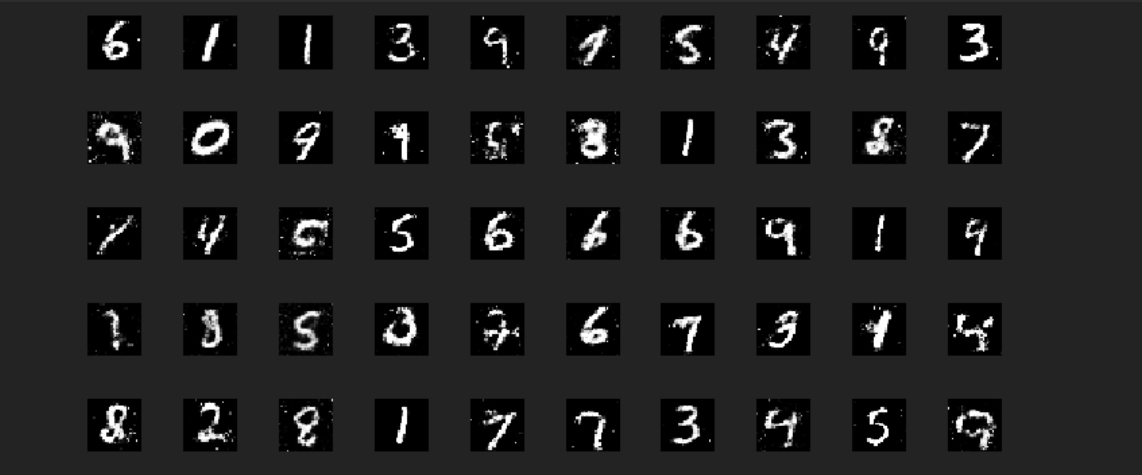Congratulations!! You have successfully implemented your first GAN model.

Visit the google developers’ documentation of GANs to learn more!!

This site uses Akismet to reduce spam. Learn how your comment data is processed.